Next: Reduction to ecliptic Up: Lunar motion Previous: Evection

# Evection in latitude

Next, let us consider terms in the solution of the lunar equations of motions that depend linearly on the lunar inclination,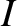.

According to Equation (11.155),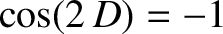(11.305)

It follows from Equations (11.209), (11.213), and (11.217) that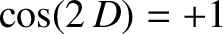(11.306)

According to Equation (11.156),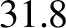(11.307)

It follows from Equations (11.209), (11.214), and (11.217) that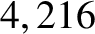(11.308)

According to Equation (11.152),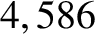(11.309)

It follows from Equation (11.217), as well as the previous expression for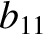, that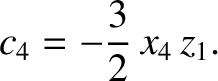(11.310)

The preceding expression can be combined with Equation (11.208) to give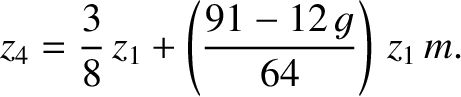(11.311)

The non-trivial solution of this equation is such that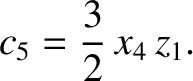(11.312)

It follows from Equation (11.210) that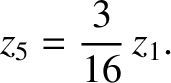(11.313)

Thus, setting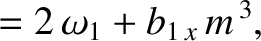, we get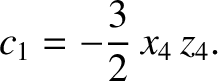(11.314)

The arbitrary parameter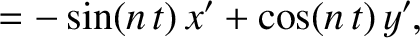is chosen such that the parameter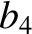, appearing in Equation (11.124), is the same as in the undisturbed motion. Thus, making use of Equation (11.184),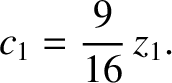(11.315)

Hence, Equations (11.306) and (11.308) reduce to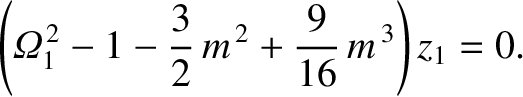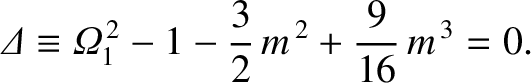(11.316) and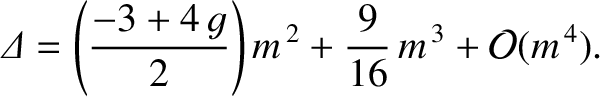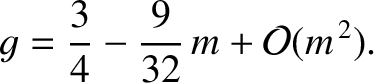(11.317)

It follows from Equations (11.122)-(11.124), (11.184), (11.187), and (11.188), as well as the previous expressions for,, and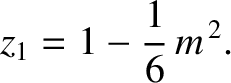, that the net perturbation to the lunar orbit due to terms in the solution of the lunar equations of motion that depend linearly onis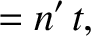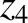(11.318)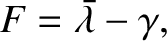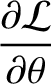(11.319) and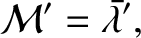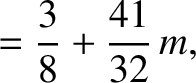(11.320)

The previous expressions are accurate to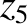.

The first term on the right-hand side of expression (11.320) is Keplerian in origin (i.e., it is independent of the perturbing influence of the Sun). The second term, which is known as evection in latitude, is due to the combined action of the Sun and the inclination of the lunar orbit to the ecliptic. Evection in latitude can be thought of as causing a slight increase in the inclination of the lunar orbit at the times of the first and last quarter moons (i.e., when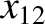and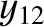), and a slight decrease at the times of the new moon and the full moon (i.e., when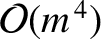and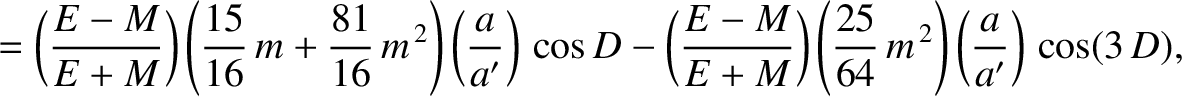). (See Exercise 4.) Evection in latitude generates a perturbation in the lunar ecliptic latitude that oscillates sinusoidally with a period of 32.3 days, and has an amplitude (calculated with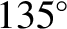and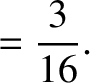) of 602 arc seconds. As before, the oscillation period is in good agreement with observations, but the amplitude is somewhat inaccurate [it should be 624 arc seconds (Chapront-Touzé and Chapront 1988)] due to the omission of higher order (inand) contributions.Next: Reduction to ecliptic Up: Lunar motion Previous: Evection
Richard Fitzpatrick 2016-03-31